info@biomedres.us   +1 (502) 904-2126   One Westbrook Corporate Center, Suite 300, Westchester, IL 60154, USA   Site Map

Impact Factor : 0.548

# Measurement of Density, Refractive Index and Conductance of Some Pyrimidine Derivatives in Solutions at 308.15 K. Volume 7 - Issue 4

Shipra Baluja*, Rahul Bhalodia and Ravi Gajera

• Department of Chemistry, University of Saurashtra, India

Received: July 16, 2018;   Published: August 03, 2018

*Corresponding author: Shipra Baluja, Department of Chemistry, University of Saurashtra, Rajkot-360005, Gujarat, India

DOI: 10.26717/BJSTR.2018.07.001532

## Abstract

Some new pyrimidine derivatives have been synthesized and their physicochemical parameters such as density, refractive index and conductance have been measured in N,N-dimethyl formamide and tetrahydrofuran at 308.15 K. It is observed that solvent and structure of compounds have major effect on these studied properties which may be due to different type of interactions in different solvents for different compounds.

Abbreviations: Density; Refractive Index; Conductance; N, N-Dimethyl Formamide; Tetrahydrofuran

## Introduction

Among many heterocyclic compounds, pyrimidine compounds are one of the most prominent structures which attract many chemists and pharmacists due to their therapeutic values [1-10]. The applications of these compounds in drug research have stimulated the invention of wide range of synthetic methods for their preparation and chemical transformations [11-15]. Further, many of these compounds are known to exist in deoxyribonucleic acid and ribonucleic acid which is one of the most essential constituents of all cells and thus of all living matter. As many of these compounds exist in various drugs [16-18], it would be useful to study their physical parameters which are prime factors for the study of these compounds in pharmaceutical field [19-21]. Thus, in the present work, physicochemical parameters such as density, refractive index and conductance of some newly synthesized pyrimidine derivatives such as dihydropyrimidine and dihydropyrimidinthiones have been measured in N,N-dimethyl formamide, dimethylsulphoxide and tetrahydrofuran at 308.15 K.

### Experimental>

Some new dihydropyrimidine and dihydropyrimidinthiones compounds have been synthesized. The general structures and substitutions in different compounds are given in Figure 1.

### pysicochemical Studies>

The solvents DMF, DMSO and THF used for physicochemical studies were purified by fractionally distillation by the reported method . For each compound, a series of solutions of different concentrations were prepared in these solvents. The choice of different solvents for dihydropyrimidine and dihydropyrimidinthiones is due to solubility problem. The density and refractive index of pure solvents and solutions were measured by using pycnometer and Abbe refractometer respectively at 308.15 K. The temperature was maintained by circulating water through jacket around the prisms of refractometer from an electronically controlled thermostatic water bath (NOVA NV-8550 E). The uncertainty of temperature was ± 0.1oC. The conductance of each solution was measured by using Elico Conductivity Meter (Model No. CM 180) at 308.15 K. The measured conductance was corrected by subtracting the conductance of pure solvent.

## Results and Discussion

Table 1 shows the experimental values of densities and refractive index for all the studied solutions. Using experimental density of solution, density of each compound was calculated using the following relation:

where ρ12 is the density of solution and ρ1 and ρ2 are the densities of solvent and solute respectively. g1 and g2 are the weight fractions of solvent and solute.

The evaluated densities of all the compounds are listed in (Table 2) along with the theoretical densities which were calculated using the following equation :

Table 1: The density (ρ12) and refractive index (n) of synthesized compounds at 308.15K.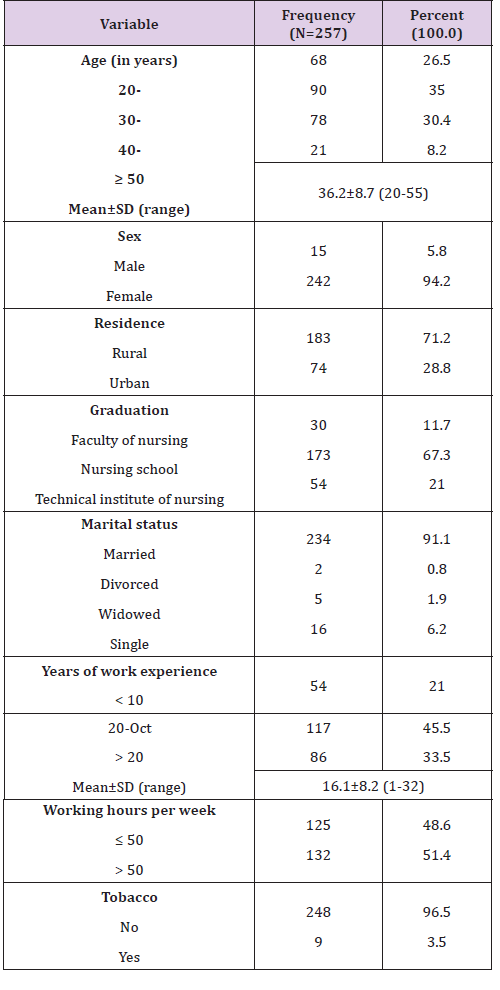Table 2: Experimental and theoretical densities of compounds at 308.15 K.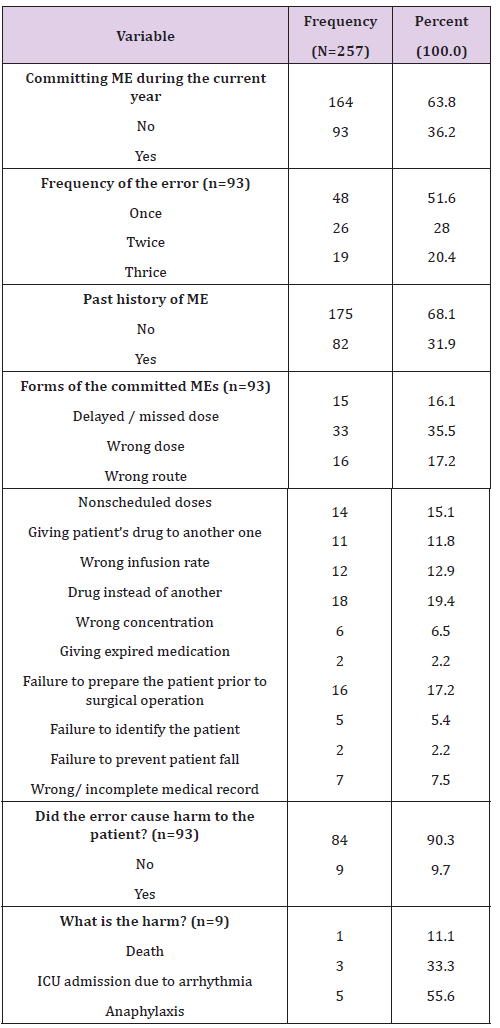where ρ is the density of the compound, K is packing fraction (0.599), M is the molecular weight of the compound, NA is the Avogadro’s number and ΔVi is the volume increment of the atoms and atomic groups present in the compound.

Comparison of densities values showed that theoretical density values are different from those evaluated from experimental data. Further, for the same compound, density in the two solvents is different. This suggests that solvent plays an important role. In solutions, compounds interact differently depending upon their substitution, structure and nature of solvent. These molecular interactions affect volume which causes change in density.

Further, the molar refraction of a pure liquid (MRD)1 were calculated by the following equation:

For solutions, the following equation was used to determining molar refraction:

where n12 and ρ12 are refractive index and density of solution respectively. X1 and X2 are the mole fractions and M1 and M2are the molecular weight of the solvent and solute respectively

From the values of the molar refraction of solution and pure solvent, molar refraction of solid compounds was determined by following equation:

From the density and molar refraction data, the refractive indexes of all the compounds were calculated from equation (5). The molar refraction (MRD)2 and refractive index of all the compounds are reported in Table 3 for 0.1M solution.

It is evident from Table 3 that both (MRD)2 and refractive index of compounds are different in each solvent. This again proves that in different solvents, intermolecular interactions are different, which affect these parameters. In some solvents, there may be interaction between solute and solvent molecules where as in others breakage of bonds may take place. As refractive index and molar refraction depends not only upon atomic refraction but also upon single, double or triple bonds, these parameters are affected by the type of interactions taking place in solution. Further, bond polarity also causes change in molar refraction. Thus, type of solvent affects the refractive index and molar refraction of a solute. The measured conductance (k) of each solution after correction is listed in Table 4. It is observed that for all the studied compounds, conductance increases with concentration in all the solvents. For dihydropyrimidinthiones compounds, conductance is lower in THF than that of DMF which may be due to greater electro relaxation effect owing to the higher permittivity of THF, which contributes interionic repulsions to a larger extent . For dihydropyrimidine, conductivity is less in DMSO than that in DMF.

Table 3: Molar refraction (MRD)2 and refractive index (n) of 0.1 M solutions of compounds at 308.15 K.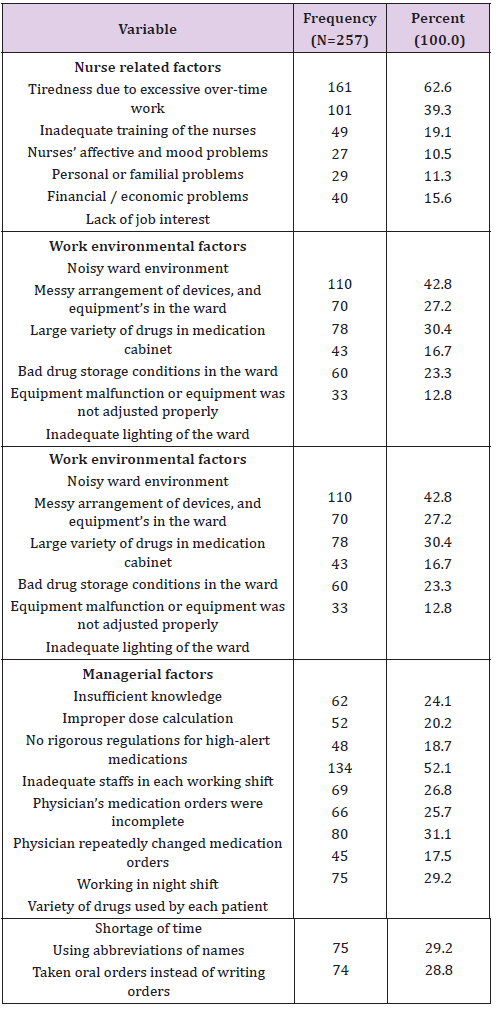Table 4: Molar refraction (MRD)2 and refractive index (n) of 0.1 M solutions of compounds at 308.15 K.From corrected conductance, specific conductance (κ) and equivalent conductance (λc) are calculated using the following equations:

where θ is the cell constant (0.96 cm-1) and c is the concentration (g. equi. /lit.) of solution.

Table 5: The limiting equivalent conductance (λ0) of some synthesized compounds at 308.15 K.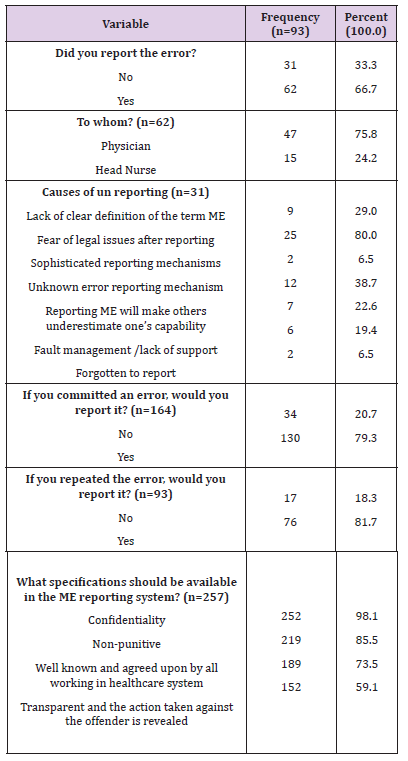The equivalent conductance (λc) is plotted against √C for all compounds as shown in (Figures 1 & 2). For all the dihydropyrimidinthiones and dihydropyrimidine, the equivalent conductance increases with concentration in both the solvents. It is obvious from figures that some of the compounds behave as strong electrolytes whereas others exhibited weak electrolytic behavior. The behavior is different in different solvents. For weak electrolytes, it is difficult to determine λ0. However, for some of the compounds in the studied solutions of compounds, λ0 values are evaluated approximately by extrapolation method and are reported in Table 5.

Figure 1: General structure of synthesized different pyrimidine derivatives.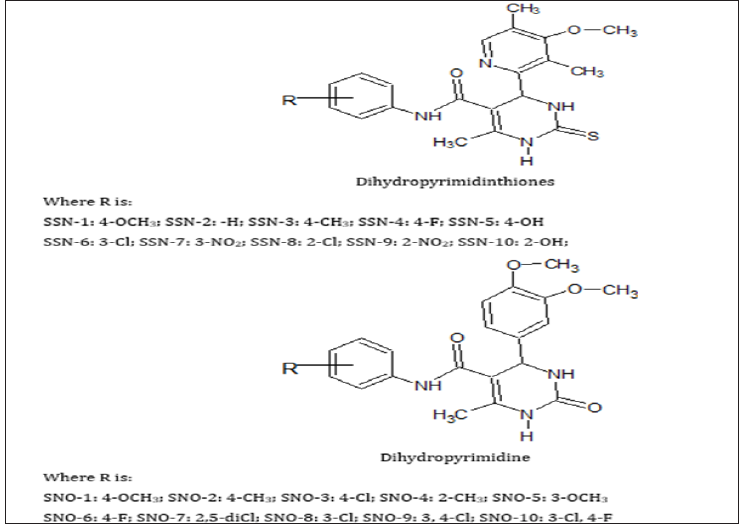Figure 2: The variation of equivalent conductance with C for Dihydro pyrimidinones in [A] DMF and [B] DMSO at 308.15 K.## Conclusiont

It is observed that physicochemical parameters of compounds in solution depends not only on the structure and substitution of the compound but also on the nature of solvent in which it is dissolved. The molecular interactions occurring in the solution affect volume which in turn causes a small change in density and refractive index. Depending upon the nature of solvent, the conductance i.e., electrolytic behavior of compounds also changes.